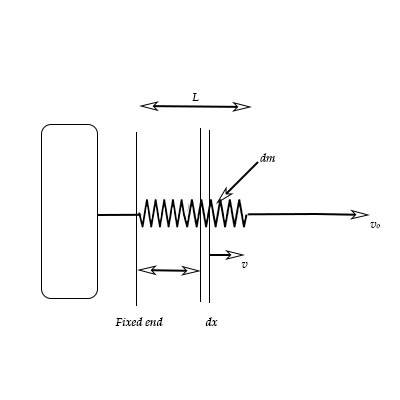# Consider a small segment dx of a spring with a mass dm located a distance x away from the fixed...

## Question:

Consider a small segment {eq}dx {/eq} of a spring with a mass {eq}dm {/eq} located a distance {eq}x {/eq} away from the fixed end of the spring.

Determining the effective mass of the spring that contributes to the kinetic energy of the mass-spring system. The kinetic energy of this segment is given by:

{eq}dK = \frac {1}{2} v^2dm {/eq}

where {eq}v {/eq} is the speed of the small segment of spring and depends on the position {eq}x {/eq} along the spring (i.e. v = {eq}v(x) {/eq}.

This is because the right end of the spring is moving at full speed ({eq}v = v_0 {/eq} at {eq}x = L {/eq}) while the left end isn't moving at all ({eq}v = 0 {/eq} at {eq}x = 0 {/eq}). Assuming the spring has a uniform mass per unit length {eq}\lambda = m_{SPRING}/L, {/eq} show that the spring contributes only one-third of its mass when calculating the kinetic energy. That is {eq}K_{SPRING} = \frac {1}{2} (\frac {1}{3} m_{SPRING}) v^2_0. {/eq}## Kinetic energy:

Kinetic energy can be defined as the type of energy that gets produced in any object due to the motion. The elastic object that collects the energy within it, while it is pressed or expanded is known as spring.

Given data

• The small segment of spring is dx.
• The mass of segment is dm.
• The distance of segment from end is x.
• The kinetic energy of segment is {eq}dK = \dfrac{1}{2}{v^2}dm {/eq}.
• The uniform mass per unit length is {eq}\lambda = \dfrac{{{m_{spring}}}}{L} {/eq}.

As, it is given that mass density is {eq}\lambda = \dfrac{{{m_{spring}}}}{L} {/eq}.

But,

{eq}\lambda = \dfrac{{dm}}{{dx}} {/eq}

So, it can be written as,

{eq}dm = \dfrac{m}{L}dx {/eq}

The kinetic energy of segment is given,

{eq}dK = \dfrac{1}{2}{v^2}dm......\left( 1 \right) {/eq}

Substituting the values in above expression and integrating,

{eq}\begin{align*} dK &= \dfrac{1}{2}{v^2}\dfrac{m}{L}dx\\ K &= \dfrac{1}{2}\int {{v^2}\dfrac{m}{L}} dx......\left( 2 \right) \end{align*} {/eq}

The velocity of mass element can be given as,

{eq}v = \dfrac{{{v_o}x}}{L} {/eq}

Therefore, equation 2 becomes,

{eq}\begin{align*} K &= \dfrac{1}{2}\dfrac{m}{L}\int_0^L {\dfrac{{v_o^2{x^2}}}{{{L^2}}}} dx\\ K &= \dfrac{1}{2}\dfrac{{mv_o^2}}{{{L^3}}}\left( {\dfrac{{{x^3}}}{3}} \right)_0^L\\ K &= \dfrac{1}{2}\dfrac{{mv_o^2}}{{{L^3}}} \times \dfrac{{{L^3}}}{3}\\ K &= \dfrac{1}{2}\left( {\dfrac{1}{3}{m_{spring}}} \right)v_o^2 \end{align*} {/eq}

Thus, the spring contributes only one-third of its mass when calculating the KE.